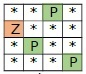# Find safe cells in a matrix in C++

Suppose we have a matrix mat[][]. It has character Z and P. The Z is the zombie and P is the plant. And another character * is a bare land. A zombie can attack the plant, when plant is adjacent to zombie. We have to find number of plants, that are safe from zombie. Suppose the matrix is like below −So there are only two plants that are safe.

We will traverse the matrix element by element, then when the current element is a plant, then check that the plant is surrounded by zombie or not, if not then increase the count.

## Example

Live Demo

#include<iostream>
using namespace std;
bool isZombie(int i, int j, int r, int c, string mat[]) {
if (i < 0 || j < 0 || i >= r || j >= c || mat[i][j] != 'Z')
return false;
return true;
}
int countSafeCells(string matrix[], int row, int col) {
int i, j, count = 0;
for (i = 0; i < row; i++) {
for (j = 0; j < col; j++) {
if (matrix[i][j] == 'P') {
if (!isZombie(i - 1, j - 1, row, col, matrix) && !isZombie(i - 1, j, row, col, matrix)
&& !isZombie(i - 1, j + 1, row, col, matrix) && !isZombie(i, j - 1, row, col, matrix)
&& !isZombie(i, j, row, col, matrix) && !isZombie(i, j + 1, row, col, matrix)
&& !isZombie(i + 1, j - 1, row, col, matrix) && !isZombie(i + 1, j, row, col, matrix)
&& !isZombie(i + 1, j + 1, row, col, matrix)) {
count++;
}
}
}
}
return count;
}
int main() {
string mat[] = { "**P*", "Z***", "*P**", "***P" };
int row = sizeof(mat) / sizeof(mat);
int col = mat.length();
cout << "Number of safe cells: " << countSafeCells(mat, row, col);
}

## Output

Number of safe cells: 2